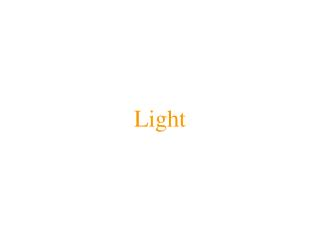DownloadDownload PresentationLight

# Light

Download Presentation## Light

- - - - - - - - - - - - - - - - - - - - - - - - - - - E N D - - - - - - - - - - - - - - - - - - - - - - - - - - -
##### Presentation Transcript

1. Light

2. Intensity calculation  = wavelength I() = wavelength  intensity of light reaching eye I() = Idiff() + Ispec() + Irefl() + Itrans() + Iamb(). Idiff() = diffuse component of I() Ispec() = specular component of I() Irefl() = reflected light component of I() Itrans() = transmitted light component of I() Iamb() = ambient component of I()

3. Diffuse reflection • Idiff() = diffuse component of I(). • Idiff() = kdiffj Sj ILj() Fdiff() (N • Lj). • kdiff = diffuse reflectance coefficient; • Sj = light j shadow coefficient (0 = shadow; 1= no shadow); • ILj() = intensity of light j; • Fdiff() = diffuse reflection curve (object color); • N = surface normal; • Lj = light direction for light j.

4. Specular reflection • Ispec() = specular component of I(). • Ispec() = kspecj Sj ILj() Fspec() (N • Hj)f. • kspec = specular reflectance coefficient; • Sj = light j shadow coefficient (0 = shadow; 1= no shadow); • ILj() = intensity of light j; • Fspec() = specular reflection curve (white); • f = specular exponent; • N = surface normal; • Hj = vector halfway between viewing direction and light. • Hj = where V and Lj are the viewing and light directions.

5. Ambient light • Iamb() = ambient component of I(). • Iamb() = kamb Ea() Famb(). • kamb = ambient coefficient; • Ea() = ambient light intensity of environment; • Famb() = ambient reflection curve (usually Famb() = Fdiff()).

6. Reflection from other surfaces • Irefl() = reflected light component of I(). • Irefl() = kspecI(R*, ) Fspec() A(|R*|). • kspec = specular reflectance coefficient; • I(R*, ) = intensity of wavelength  in reflection ray R*; • Fspec() = specular reflection curve (usually white); • A(|R*|) = distance attenuation of reflection ray R*; • R* = reflection ray; • |R*| = length of reflection ray R*; • R = reflection direction. • R = –V + 2 (N • V) N where N and V are the normal and view directions.

7. Transmitted light • Itrans() = transmitted light component of I(). • Itrans() = ktransI(T*, ) Fspec() A(|T*|). • ktrans = transparency coefficient (0 = opaque; 1 = transparent); • I(T*, ) = intensity of wavelength  in transmitted ray T*; • Fspec() = specular reflection curve (usually white); • A(|T*|) = distance attenuation of transmitted ray T*; • T* = transmitted ray; • |T*| = length of reflection ray T*; • T = transmitted ray direction. • T = (1/ 2)(–V) + ((1/ 2)cos(1) – cos(2)) N.

8. Snell’s Law • N = normal direction; • V = view direction; • T = transmitted ray direction; • 1 = refraction index for material 1 (V and N point to material 1); • 2 = refraction index for material 2 (T points to material 2); • 1 = angle of incidence (cos(1) = NV); • 2 = angle of refraction (cos(2) = (–N)T). • Snell’s law:

9. Transmitted ray direction • T = transmitted ray direction. • T = (1/ 2)(–V) + ((1/ 2)cos(1) – cos(2)) N • = (1/ 2)(–V) + ((1/ 2)(NV) – cos(2)) N. • where: • N = normal direction; • V = view direction; • 1 = refraction index for material 1 (V and N point to material 1); • 2 = refraction index for material 2 (T points to material 2); • 1 = angle of incidence; • 2 = angle of refraction. • cos(2) =

10. Refraction Index • Vacuum: 1; • Glass (crown): 1.52; • Glass (dense flint): 1.66; • Water: 1.33; • Fused quartz: 1.46.

11. Cook-Torrance lighting model • Ispec() = specular component of I(). • Ispec() = • F(, ) = Fresnel term; • = half of angle between V and Lj = angle between Hj and Lj; • D(m,) = density of microfacets in direction Hj; •  = angle between Hj and N; • m = surface roughness (between 0 and 1); • G(N,V,Lj) = masking and shadowing term; • V = viewing direction; • Lj = light direction for light j; • N = surface normal; • Hj = vector halfway between V and Lj.

12. Fresnel term • F(, ) = Fresnel term. • where: • c = cos() = Lj Hj; • g2 = 2 + c2 – 1; •  = index of refraction at wavelength . • When  = 0:

13. Density and masking terms • D(m, ) = density of microfacets in direction Hj. • m = surface roughness (between 0 and 1.) • G(N,V,Lj) = masking and shadowing term.